A Quantum Primer

Introduction.

This little book discusses key experiments and ideas at the root of quantum mechanics. Its intended audience is students contemplating a first course in the subject. The dual goals are (a) to create a conceptual framework into which the student can fit the mathematics that will be encountered in a first course, and (b) to provide the prospective student with a sample, however brief, of the mathematical nature of the theory.

The early evolution of the theory is traced up through a statement of the Schroedinger equation. The many ramifications of that seminal equation … both mathematical and theoretical … are left for formal course work.

Quantum theory constitutes a clear break with many cherished and even axiomatic ideas of the classical era. For example, classically it is assumed as a matter of course that the energy of a system can assume any of a continuum of values. The discovery that energy (and other quantities) actually assume only discrete values not only marked the beginnings of a new theory of matter and light, but initiated a search for new ways of thinking that continues to the present day.

The quantum view of the world requires nothing less than a philosophical shift in one’s thought processes. If this primer facilitates such a shift, then it will have fulfilled the author’s intent.

1. The Quantization of Energy.

Every isolated material body whose temperature is above absolute zero emits heat radiation. If it absorbs radiant energy at the same rate as it radiates energy then its temperature remains constant.

Most bodies also reflect part of any incident radiation, and it is not always possible to discriminate between radiated and reflected radiation. A special exception is so-called black bodies. These completely absorb all incident radiation, and thus all emitted radiation is heat radiation.

If an absorption coefficient, a(l), is defined such that the fraction of absorbed radiation in wavelength range l to l+dl (or (l,dl) for short) is a(l)dl, then in the case of a black body a(l)=1.

A body’s emissive power, R(l,T), is defined such that the amount of heat radiation emitted per unit area (per unit time) in the range (l,dl) at temperature T is R(l,T)dl. In 1859 Kirchoff proved (using thermodynamic arguments) that the ratio R(l,T)/a(l) is the same for all materials. And of course R(l,T) has its maximum value for black bodies (where a(l)=1). The total emissive power for a black body is just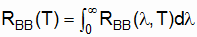(1_1)

In 1879 Stefan found empirically that RBB(T) is proportional to T4: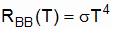(1_2)

Boltzmann later derived the same result using thermodynamic theory, and Eq. 1_2 is now known as the Stefan-Boltzmann law. (s = 5.67E-8 Watt/meter2/K4 is known as Stefan’s constant.)

A practical example of a black body is a blackened cavity (of any material) linked to the external environment by a small hole. Virtually all of the radiation entering the hole is absorbed by the cavity walls. Thus any radiation coming out of the hole qualifies as black body radiation. This radiated power can be measured, and division by the hole’s cross section then gives an empirical measurement of RBB(l,T). In 1899 Lummer and Pringsheim measured and plotted RBB(l,T) vs. l at a number of different temperatures. The plot was always a lopsided Gaussian, falling toward zero as l went to zero and infinity, and peaking at values of l that decrease as T is increased.

The emissive power emanating from the hole is a measure of the radiant energy density in the cavity: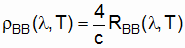. (1_3)

Thus the Lummer-Pringsheim measurements of RBB(l,T) also provide experimental values for rBB(l,T). The radiant energy density is usually referred to as the spectral distribution function.

There was a good deal of interest in deriving a formula for rBB(l,T) from existing theory. Wien had shown theoretically, in 1893, that rBB(l,T) had to be of the form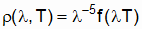, (1_4)

where f is an unknown function of the "single" variable lT. But what was needed was an explicit formula for rBB(l,T).

Rayleigh and Jeans theorized that the energy in the cavity was absorbed and emitted by microscopic dipole oscillators in the cavity walls. It was generally believed that the oscillation frequencies of such dipoles could range continuously from zero to infinity, and that the range of amplitudes (and thus of energies) at each frequency was also continuous. Among other things this implied that the energies of emitted (and absorbed) electromagnetic waves could (in theory) range continuously from zero to infinity.

Rayleigh and Jeans reasoned that the radiation in the cavity must be in the form of standing waves, with nodes at the walls. For cavity dimensions that are large compared to the wavelengths, they were able to show mathematically that n(l)dl, the number of different waves (or "modes") per unit volume in range (l,dl), must be proportional to l-4: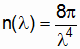. (1_5)

If |E(l,T| is the mean energy in the modes of wavelength l, at temperature T, then the spectral distribution function must be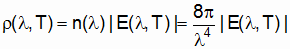.(1_6)

In order to determine a formula for |E(l,T)|, Rayleigh and Jeans invoked the Equipartition Theorem for the dipole oscillators in the walls. According to that theorem the average energy of the set of oscillators associated with r(l,T)dl is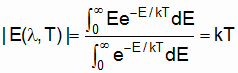. (1_7)

Since the oscillators are constantly exchanging energy with the cavity radiation, kT should also be the average energy of each mode (or standing wave) in the cavity. Thus according to Eq. 1_6 the energy density in (l,dl) should be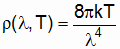. (1_8)

An objection to the Rayleigh-Jeans formula is that it does not agree with the less explicit result (Eq. 1_4) derived by Wien. A more glaring theoretical objection is that the formula for r(l,T) goes to infinity as l goes to zero. Clearly we would not expect such immense energy densities in any real, finite cavity. Most objectionable of all was the disagreement with the values of r(l,T) measured by Lummer and Pringsheim. Whereas the Lummer-Pringsheim curve goes to zero as l goes to zero, the Rayleigh-Jeans formula shoots off toward infinity. The physics community was so convinced of the essential correctness of the Equipartition Theorem that this disagreement of Eq. 1_8 with the experimental data was dubbed "the ultraviolet (i.e., short wavelength) catastrophe!"

In 1900 Planck found (reportedly by trial and error) that the following formula for r(l,T) fits the experimental data of Lummer and Pringsheim: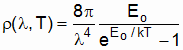. (1_9)

In this formula Eo is the smallest nonzero amount of energy that can exist in modes with wavelengths in (l,dl). In order to derive this formula from "first principles," Planck had to assume that the energy of a radiation mode (and/or of the associated oscillators in the cavity wall) could not assume a continuum of energies, but could only have discrete energies in the amounts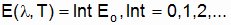(1_10)

Since E is not continuous, the Equipartition Theorem integrals (Eq. 1_7) must be replaced by sums: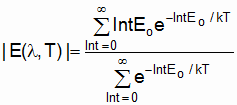. (1_11)

In order to satisfy Wien’s law (Eq. 1_4), Eo had to be inversely proportional to l: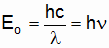. (1_12)

In this case Eq. 1_9 becomes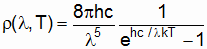. (1_13)

Planck’s constant, h=6.63E-34 joule seconds, is very small and thus the quantization of energy is not evident in macroscopic cases. However, it appeared to be essential in fitting the Lummer-Pringsheim data. Planck reportedly was never comfortable with the energy quantization idea, perhaps partly because the idea that the radiant energy in the cavity was, by implication, also quantized … a result that seems to imply quantization of the field vectors.

Einstein, on the other hand, theorized that the quantization of radiant energy is generally true … an idea that explained several unclassical aspects of the photoelectric effect. Einstein also seized on the oscillator energy quantization to explain anomalies in the specific heats of solids at low temperatures. As the quantization-of-energy concept gained acceptance, other physical quantities would also be quantized (notably the quantization of angular momentum suggested by Bohr).

2. Photoelectricity.

As early as 1887 Hertz produced electromagnetic waves by causing sparks to jump between electrodes at high potential differences. He found that the passage of sparks was mysteriously facilitated when the electrodes were illuminated with ultraviolet light.

Hallwachs, Stoletov and Lenard subsequently found that, even without the creation of sparks, charged particles are emitted by metallic surfaces that are illuminated by short wavelength electromagnetic waves. Using techniques similar to those developed by Thomson in his discovery of the electron, Lenard was able to measure the charge-to-mass ratio of the expelled particles and demonstrated that they were probably electrons. Somehow the incident light was exciting electrons in the metallic surface and causing them to jump out of the metal.

If a second, non-irradiated surface is placed nearby, and if a circuit-closing wire connects the two surfaces, then some of the ejected electrons are captured on the non-irradiated surface and a small current flows. This "photoelectric" current can be amplified by holding the ejecting surface at a slightly negative potential, and the collecting surface at a slightly positive potential. The potential difference is not nearly enough to cause sparks. But the slight potential difference ensures that more of the electrons, ejected by the incident light, find their way to the non-irradiated electrode. Not surprisingly, the magnitude of the current increases as the intensity of the radiation on the emitting surface is increased.

Lenard was able to drive the current to zero by reversing the polarity slightly, making the collecting surface negative. In this case practically all of the ejected electrons are drawn back into the irradiated surface. He found that, at a given wavelength of the irradiating light, there is a definite stopping potential, Vo, at which all of the ejected electrons are drawn back to the irradiated surface. The puzzling feature was that this stopping potential works at all intensities of the illuminating radiation … even very large ones! That is, Vo depends only on the incident radiation’s wavelength, and not at all on its intensity. And if l is greater than a certain threshold, then no electrons are ejected no matter how intense the illuminating radiation may be!

Lenard carefully plotted the stopping potential vs. the irradiating radiation’s frequency and ended up with a linear plot. He also found that, for frequencies greater than the threshold frequency, the photoelectric current begins to flow practically immediately, regardless of how low the intensity of the illuminating radiation is.

In 1905 Einstein proposed that all of these decidedly unclassical behaviors could be explained if the energy in the illuminating radiation is "corpuscular," in the sense that it interacts with the electrons practically at points in space and time. He used Planck’s formula for the energy of one of these "photons" or "quanta of light energy":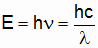. (2_1)

According to Einstein, whether or not an individual electron can break free of the irradiated surface depends only on the energy of the photon it interacts with. Increasing the intensity of the illuminating radiation might increase the number of ejected electrons, but it doesn’t increase the individual escape energies. Furthermore, some interactions occur practically instantaneously when the radiation is turned on; there isn’t a waiting period during which the presumably dispersed energy of classical waves must build up.

Einstein theorized that a certain, minimum amount of energy would be needed for a photoelectron to break free of the irradiated surface. He termed this amount of energy "the work function" (W for short). The maximum kinetic energy of escaped electrons would then equal the incoming photon energy minus the work function: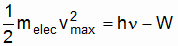. (2_2)

Eq. 2_2 is known as Einstein’s equation. It was verified in a series of delicate experiments by Millikan.

No one was sure of just how the irradiating photons interact with the ejected electrons. Did the photons somehow pull the electrons out of the irradiated metal? Or did they drive them deeper into the metal, where they collided elastically with lattice atoms and then recoiled out of the metal with kinetic energies greater than W? Less than a quarter century later Compton would show that the latter was the most probable mechanism. Like material particles, Einstein’s light corpuscles (or photons) were evidently elastically colliding with loosely bound conduction electrons!

3. The Duane-Hunt Rule.

According to Einstein, when energetic photons smash into a metallic surface’s electrons, the electrons can be knocked right out of the surface. In this section we discuss the emission of photons when electrons smash into matter.

Let us suppose that electrons are initially accelerated through a voltage, V. Their kinetic energy is eV, where e is the electron’s charge. A beam of such energized electrons is allowed to smash into a grounded sample of some element (typically Molybdenum). At least two types of collision seem plausible: (1) electrons collide with entire atoms that are locked in the sample’s atomic lattice; (2) electrons collide with atomic electrons in the target material.

Let us first consider the collision of an electron with an entire atom (or, for that matter, with the entire lattice of atoms). The electron enters the material, bounces off a virtually immovable atom, then perhaps bounces off another one, etc. With each collision the electron very slightly heats up the sample ("very slightly" owing to the electron’s minuscule mass). But unlike photons, electrons are charged. And with each collision some amount of radiation is emitted … how much depends upon the impact parameters. Given a beam of many electrons bombarding the sample, a continuous range of wavelengths is emitted.

Of course there may be some electrons whose first collision is "dead on." They are brought practically to rest in the sample after the first collision. In these cases virtually all of the electron’s initial kinetic energy is converted to a photon: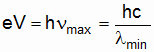. (3_1)

Eq. 3_1 is known as the Duane-Hunt rule. It has been corroborated experimentally using diverse target materials.

The second type of collision is when an incident electron collides with an atomic electron. Here the energies of both electrons may be significantly altered. The suggested mechanism is: (1) incoming electron gives DE of its energy to an atomic electron; (2) a photon gets generated when the atomic electron reverts to its original energy (for reasons unknown). Note in this case that all or part of the incoming electron’s kinetic energy is not immediately converted to a photon. Rather the kinetic energy is converted to a greater energy for the bound, atomic electron. The energy is then emitted as a photon when the excited atomic electron "drops back" to its original state.

As in the whole-atom collision case, we might classically expect that, collectively, a continuum of wavelengths would be generated. But somewhat mysteriously it turns out that only sharply defined wavelengths are generated. It is as if only highly selective energy exchanges can occur. These discrete DE’s are characteristic of the target material. If DE is an incoming electron’s loss of kinetic energy in one of these collisions, then a la Duane-Hunt we expect the finally emitted photon wavelength to satisfy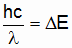. (3_2)

But why should atomic electrons be so picky about what amounts of energy they will accept from bombarding electrons? And what was the rule that specifies just what these discrete energies might be in a given element’s case? Neils Bohr wondered about such matters.

4. Atomic Spectra and the Bohr Atom.

While Einstein and others were pondering the ramifications of quantized energies in radiation and matter, others were busy measuring the frequencies of light from pure elements. The general procedure was to subject a gaseous element to an electric spark or flame. Unlike the continuous range of wavelengths emitted by solids at temperatures above absolute zero, only discrete wavelengths are emitted when gaseous elements are so energized. And just as the atomic electrons of a solid appear to be highly selective about the energies they will accept during collisions with free electrons, so did the atoms of a gaseous element appear to be choosy about what energies they would absorb and subsequently emit. Indeed it was found that a given element both absorbs and emits the same discrete amounts of energy, and for unknown reasons eschews all others.

By the turn of the century a good deal of data had been collected on the series of discrete wavelengths for the various elements. Balmer, and later Rydberg worked out formulas that fit these series, but the theoretical explanation for such atomic selectivity and orderliness remained a mystery. Indeed the very structure of atoms was a matter of conjecture and little more.

Around 1912 Rutherford and his students, Geiger and Marsden, attempted to learn something about atomic structure by bombarding metal foils with alpha particles (Helium nuclei). By measuring the deflection of such doubly charged particles, they primarily hoped to learn something about the distribution of positive charge in an atom. (The existing, but unproved Thomson model theorized that the atomic positive charge occupied the entire atomic space, with electrons embedded therein.) In general, owing to the alpha particle’s mass, Rutherford and his students expected the particles to be deflected little when passing through the smeared out "clouds" of positive charge.

Much to their surprise they found that some of the alpha particles were deflected through angles on the order of 180o. They felt obliged to conclude that the atom’s positive charge is actually concentrated in a tiny "nucleus" with hitherto unsuspected charge density. This left the electrons occupying the bulk of the atomic volume.

A theoretical difficulty at this juncture was that there is no stable static arrangement of positively and negatively charged particles. In order not to sit right on top of the positively charged nucleus, the electrons would have to move. But this idea was also not without its theoretical difficulties. For any such dynamic model would theoretically result in radiation, and clearly atoms do not constantly radiate.

In 1913 Bohr advanced a "solar system" model for the Hydrogen atom in which the lone electron circles the much more massive, practically resting nucleus (proton). Bohr met the objection that such an electron should radiate by simply stating that it does not. Rather he theorized that there are stable states (or orbits) in which no radiation occurs. Radiation is absorbed and emitted when the electron transitions between such stable states.

He suggested that a hallmark of stable states is that the orbiting electron’s angular momentum is quantized in amounts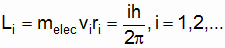(4_1)

The value of i=1 is the so-called ground state … the lowest energy state the atom can attain.

Now according to Bohr, the frequency of absorbed/emitted radiation does not equal the orbital frequency of the electron when the atom transitions from one state to another. Rather the frequency is related to the change in energy by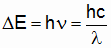. (4_2)

When the numbers were worked out, there emerged a stunning theoretical basis for the discrete spectra of gaseous elements.

In 1914 Franck and Hertz performed an experiment that elegantly demonstrates the selectivity with which atomic electrons will absorb energy from accelerating, free electrons. Because their target atoms were in a gas (typically Mercury vapor), only sharply discrete frequencies of light are emitted when the excited atoms drop back to their unexcited states. Furthermore, the emitted frequencies are consistent with Eq. 4_2. Of course people were quick to invoke Bohr’s hypothesis regarding stable states in order to explain the Franck-Hertz results. But it was still something of a mystery why the free electrons would "ignore" the target atoms (or vice versa) until the energies had risen to DE, the difference between two energy levels in the target atoms. In general, however, it was becoming clear that, at the lowest energy levels (at least), atoms and light behave in bizarre and unclassical ways.

But how could there be two physics --- one for the world we experience with our senses, and one for the unseen world of the atom (for example). Bohr suggested, with his Correspondence Principle, that there are in fact not two physics. In general, owing to the smallness of Planck’s constant, the difference between energy levels (e.g. in atoms) fades for most practical purposes into a continuum of energies as system energies grow to "macroscopic sizes." In these cases the graininess of energy transitions, etc., can be ignored and the classical laws work well. But Bohr insisted that the graininess is, in principle, always there. In brief, the new quantum theory was taking on the mantle of being the final authority in how the world works, and in particular how radiation and matter interact.

Although Bohr recognized the quantization of angular momentum (Eq. 4_1), a deeper appreciation of the reason why angular momentum (like energy) should assume only certain discrete values remained forthcoming. In 1924 deBroglie would assert that, quite as light waves exhibit particle behaviors, so do particles (like electrons) have wave characteristics! It is not difficult to show that the stable orbitals of Bohr electrons are of such size as to accommodate integral numbers of deBroglie’s electron waves.

5. The Compton Effect.

In 1923 Compton performed an experiment in which relatively free electrons were bombarded with photons. He allowed a beam of x-rays (short wavelength) to be scattered by a block of matter. The scattering of electromagnetic waves by crystals was well known. The idea was that incident waves would be reflected from crystalline planes, with no change in wavelength (so-called Thomson scattering). Furthermore, when waves are scattered from different planes, then the directions of scattering are such that the optical paths from the consecutive planes differ by integral numbers of wavelengths (so that constructive interference occurs). Here again there is no change in wavelength. The structure of crystal targets can be deduced from the observed scattering angles and knowledge of the incident light’s wavelength.

In the course of scattering x-rays off of graphite, Compton made the interesting discovery that a wavelength change occurs in the case of some of the scattered radiation. In order to explain such Dl’s, he theorized that x-ray radiation does not all simply undergo Thomson scattering. Thomson scattering, he theorized, can be expected when the x-rays are scattered from relatively massive atoms in the crystalline planes. But it occurred to him that some of the scattering might be attributable to loosely bound, practically free electrons in the graphite. According to classical theory there should again be no change in wavelength. But, convinced of the corpuscular nature of radiation, Compton wondered what would happen if an x-ray photon collided elastically with a free electron.

If two material particles collide, then the originally resting one shoots off in some direction with a newly acquired kinetic energy. And the incoming particle flies off in a different direction at a lessened kinetic energy. Compton decided that if the incoming particle was a photon, then essentially the same process might occur. But the lost energy from the incoming photon would be manifest as a gain in wavelength in the scattered photon.

Compton worked out the math of the hypothetical collision process and got near-perfect agreement with experiment. In the same year Bothe and Wilson detected the recoiled, loosely bound electrons. Two years later Bothe and Geiger found that a wave-shifted photon and the recoiling electron appear simultaneously. Finally, in 1927, Bless measured the energy of the recoiling electrons and found them to be just what Compton had predicted.

6. deBroglie’s Electron Waves.

In 1924 deBroglie suggested that, just as light (a classical wave phenomenon) behaves like particles on a microscopic scale, so might matter (a particulate phenomenon) behave like waves on a small enough scale. He suggested that, in the case of an electron, a wave’s frequency and wavelength would be related to the electron’s energy and momentum by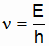, (6_1)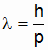. (6_2)

Eq. 6_2 had immediate appeal in the case of the Bohr Hydrogen atom. For the idea was that only orbits accommodating integral numbers of electron wavelengths could constitute stable (non-radiating) states. That is, the hypothesis was that the orbital radius must satisfy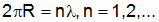(6_3)

But according to deBroglie this requires that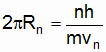, (6_4)

whence Bohr’s angular momentum quantization rule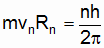. (6_5)

In 1927 Davisson and Germer "irradiated" Nickel crystals with beams of electrons, much as Bragg and others had done years earlier with beams of x-rays. (Thanks to those efforts, the spacing of Nickel crystalline planes was well known.) Davisson and Germer found that the electrons were not scattered as might classically be expected. Rather they were scattered strongly in a few select directions, and practically not at all in others. They measured the scattering angles of the post-"irradiation" electrons and calculated the "wavelengths" from the angles. Knowing the momentum of the incident electrons, they were able to corroborate Eq. 6_2.

In the same year Thomson shot electrons through thin metal foils and got the same type of diffraction patterns as the Laue patterns obtained with x-rays. Thomson’s results also corroborated Eq. 6_2.

Three years later Stern and Estermann diffracted Hydrogen and Helium atoms. In the years since, the wave properties of heavier atoms and of neutrons have been observed. In every case Eq. 6_2 has been corroborated. Evidently all particles (and not just charged particles) exhibit wave characteristics.

It was not until years later that the double slit experiment with light was duplicated with electrons. The technical problem had been to engineer slits whose width was as small as electron wavelengths. When Jonnson accomplished this feat in 1961, he observed precisely the kind of fringes that Young had produced years earlier with light. It is noteworthy that the fringe patterns are produced even when the beam of electrons is so sparsely populated that only one electron at a time, on average, passes through a slit.

A peculiar result is that the fringe pattern disappears, and the detector pattern beyond the slits is that of ultra-low wavelength particles when the slit through which each particle passes is determined. The very act of localizing a particle, as it passes through one slit or another, dramatically alters the particle wave interference pattern.

How is it that the wave nature of particles is not evident on a macroscopic scale? If the wavelength of a mote of dust is calculated, it is orders of magnitude smaller than the diameter of a proton. In such cases diffraction and interference effects are unobservable. As Bohr might say, the effects are still there. But when they are too small to detect, the classical laws provide a logical (and usually simpler) alternative to the "wave mechanics."

7. The New, Wave Physics.

Despite the successes of deBroglie’s waves, it wasn’t immediately clear what was waving, or perhaps more pointedly what the physical significance of the waves was. And as to form, were the waves longitudinal or transverse, or perhaps even circularly polarized? Did every electron (or other particle) in a monoenergetic beam have a wave, or did all the particles in an "ensemble" share a common wave (as photons seem to do)?

In 1926 Born suggested a physical meaning for the new quantum wave function, Y. First of all he suggested that Y is not characteristic of a single particle, but is abstract in the following sense: given an ensemble (or set) of "identically" prepared systems, a single wave function contains all the information that can be known about each member system. If the relative position of a particle in each ensemble system is experimentally determined, then the statistical probability that a given measurement will find a particle in the differential volume element enclosing (x,y,z) at time t will turn out to be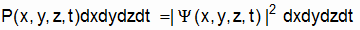. (7_1)

In other words, P is the probability density.

But if the systems are identically prepared, then why should the particles not all be at the same (relative) position some time later? The solution to this conundrum is that we cannot guarantee that all of the particles will be at some one (x,y,z) at time t=0 (for example), and that they will all simultaneously have the one momentum (px,py,pz). There is an inherent uncertainty in our simultaneous knowledge of (x,y,z, px,py,pz). Not surprisingly the passage of time may magnify this uncertainty.

The lower limit on our simultaneous knowledge of position and momentum is specified by the Heisenberg Uncertainty Relations: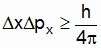, (7_2a)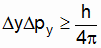, (7_2b)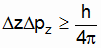. (7_2c)

The more accurate our knowledge of a particle’s x-coordinate is, the less precise our knowledge of its x-component of momentum, etc. In other words, a nonzero Dx means the particle could be anywhere in a range of x values. Similarly for the components of momentum. It is the function of |Y|2 to tell us, in a great number of observations, what fraction of the time we will find the particle "at" (i.e. in the differential volume around) x, etc. It is noteworthy that there is also a wave function (or probability density) for the particle to have one momentum or another. The "volume" enclosing px, etc., in this case would be in momentum space (with axes px, py and pz).

Of course if we must allow that a particle’s momentum lies in a range of momenta, then we must admit, a la deBroglie, that its Y is actually the superposition of a set of Yi with wavelengths li. And when a continuous range of l’s are superimposed, we end up with a wave group (or pulse). The width of this group is related to our uncertainty in particle position. And the width of a group of the momentum function is related to our uncertainty in the momentum.

Let us consider an extreme case where px is known with complete precision. Then Dx is infinite … a given observation could find an ensemble particle anywhere on the x-axis. In effect the width of the wave group is infinite, and there is a single wavelength. (Remember, in a finite-width wave group there is a range of wavelengths.)

If we know x with absolute precision, then it is px that could have any value. In momentum space we could find the particle anywhere on the px-axis. The width of the associated wave group is infinite, and the momentum wave function has a single wavelength.

8. Waves.

Let us suppose that some scalar "D" varies sinusoidally along the x-axis. We would like it to repeat every "wavelength", l. Its formula is then

D(x)=Dmaxsin(2px/l)    (8_1a)

or

D(x)=Dmaxcos(2px/l).    (8_1b)

Note that 2px/l is dimensionless (as of course it must be). D has a maximum positive value at x=p/2, p/2+2p, p/2+4p, etc. in Eq. (8_1a), and at x=0, 2p, 4p, etc. in Eq. 8_1b. A more compact notation occurs if we define the wave number, k, such that

k=2p/l. (8_2)

Then Eqs. 8_1a and 8_1b can be rewritten as

D(x)=Dmaxsin(kx), (8_3a)

D(x)=Dmaxcos(kx). (8_3b)

Now If either of these curves slides along in the positive x-direction at constant speed v, then D is a function of both x and t:

D(x,t)=Dmaxsin(kx-kvt), (8_4a)

D(x,t)=Dmaxcos(kx-kvt). (8_4b)

Again, the k makes the kvt term dimensionless. In any case, since

v=ln,  (8_5)

where n is the frequency, and If we define

w=2pn,  (8_6)

then

n=w/k    (8_7)

and we can then say

D(x,t)=Dmaxsin(kx-wt), (8_8a)

D(x,t)=Dmaxcos(kx-wt). (8_8b)

Consider Eq. 8_8a. We have

dD/dx=kDmaxcos(kx-wt/k), (8_9a)

dD2/dx2=-k2Dmaxsin(kx-wt/k). (8_9b)

And of course

dD/dt=-wDmaxcos(kx-wt), (8_10a)

dD2/dt2=-w2Dmaxsin(kx-wt). (8_10b)

Evidently Eq. 8_8a is a solution of

d2D(x,t)/dx2=(1/v2)d2D(x,t)/dt2. (8_11)

Similarly for Eq. 8_8 b. Eq. 8_11 is called the wave equation for D(x,t). Both Eqs. 8_8a and 8_8b are solutions. Furthermore, any linear combination of these two equations is a solution. If v=c, then we have the wave equation for electromagnetic waves. Maxwell was astute enough to realize this, and concluded that light is an electromagnetic wave. The rest is history.

v is called the phase velocity. It is the speed at which the curve for Eq. 8_7a or 8_7b slides along in the x-direction.

Now D can represent a single variable, such as the pressure in a sound wave. Or it can be the component of a vector, say Ex (where E is the electric field). Of course in the case of the vector E we might also require plots of Ey(x,t) and Ez(x,t). Let us say, however, that Ex=0, Ez=Eocos(kx-wt) and Ey=Eosin(kx-wt). Note that Ez and Ey are p/2 out of phase. When Ez is a maximum, Ey is zero. And when Ey is a maximum, Ez is zero. Such a wave is said to be circularly polarized.

Since Ez and Ey both have the same wavelength and frequency, a useful technique is to construct (in our imagination) a yz-plane at every value of x. Each such plane would be perpendicular to and centered on the x-axis. At time t=0 and at x=0, Ey=0 and Ez=Eo. At x=l/4 (and again at t=0) Ey=Eo and Ez=0. Indeed at t=0 the tip of the vector E traces a counterclockwise spiral around the x-axis (looking in the positive x-direction).

If this spiral slides along in the positive x-direction at speed v, then t/4 later we would find Ez(0,t/4)=0 and Ey(0,t/4)=-Eo. In general, at any fixed value of x, the vector E appears to spin clockwise around the x-axis, at an angular frequency w. The wavelength is again l=2p/k and the period is again t=2p/w. And the phase velocity is again w/k.

It is interesting that the same results can be obtained by not sliding the spiral, but rather by spinning it clockwise around the x-axis. The threads still advance in the positive x-direction with a phase velocity of w/k, etc.

Let us suppose that we opt to duplicate the sliding spiral by means of a non-translating spinning spiral. How might we represent a circular wave propagating in the negative x-direction? Since everything "moves forward" in time, we might decide still to use clockwise spinning (looking in the positive x-direction). But note that if we form a spiral using right-handed threads, then the threads propagate in the negative x-direction as the spiral spins.

In conclusion, we can represent the behavior of a circularly polarized wave by using a clockwise spinning  spiral. If the wave propagates toward positive x then we would use left-handed threads. And if it propagates in the negative x-direction we would use right-handed threads. The wavelength, l, is just the x-direction spacing between adjacent threads. And the angular frequency, w, is just the spiral's spin angular frequency.

9. Complex Waves.

Sometimes it is useful to work with complex numbers. Like E in the previous section, a complex number has two "components": a Real part and an Imaginary part. Indeed every complex number can be represented in the complex plane as the "vector" sum of its components. As Fig. 9_1 depicts, the complex plane consists of a horizontal "Real" axis and a vertical "Imaginary" axis.

Figure 9_1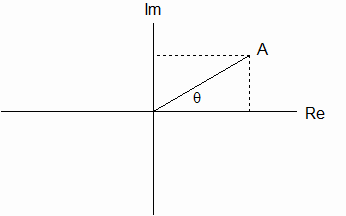Complex Number "A" in the Complex Plane

Note in Fig. 9_1 that A can be expressed as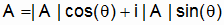. (9_1)

(When a term is preceded by "i" it means the term is Imaginary. |A| denotes the magnitude of the "vector" A in the complex plane.)

Now here is a useful mathematical identity that can be proved by expanding the series for the exponential, sine and cosine functions: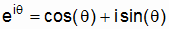. (9_2)

This being the case, Eq. 9_1 can be rewritten as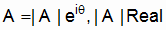. (9_3)

Note that multiplying a "vector" in the complex plane by eiq is equivalent to rotating the vector through an angle q. If q is positive, then the rotation is counterclockwise (looking down the positive x-axis); if q is negative the rotation is clockwise.

Adapting the circularly polarized wave model (last section) to complex numbers, we may define a complex wave (say Y traveling in the positive x-direction) as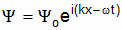. (9_4)

And we can define a complex wave traveling in the negative x-direction as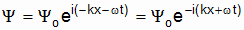. (9_5)

Like a circularly polarized real wave, Eqs. 9_4 and 9_5 appear to repeat (1) every l=2p/k in space, and (2) every t=2p/w in time. How might we visualize such complex wave functions? Let us again construct, at every value of x, a plane perpendicular to the x-axis. But this time we make it a complex plane, and put the Real axis parallel to the z-axis and the Imaginary axis parallel to the y-axis. And let us say in Eqs. 9_4 and 9_5 that Yo is real and constant. (More generally Yo could be complex and a function of x.) At x=0 (and at time t=0)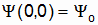. (9_6)

At x=l/4 (and at t=0)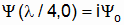, (9_7)

etc. Here again if we sit at a fixed x, say at x=0, then at time t=0 Eq. 9_6 specifies Y. But a time t/4 later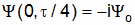. (9_8)

Notice that, looking down the positive x-axis, we have again specified an increase in x to be equivalent to a counterclockwise rotation in the complex plane(s) when the wave propagates in the positive x-direction. And we have specified an increase in time to be equivalent to a clockwise rotation around the x-axis. Evidently we can graphically think of a Y wave, propagating in the positive x-direction, as a left-handed spiral spinning clockwise around the x-axis. (If the Y wave propagates in the negative x-direction, then it has a right-handed thread with advancing x.)

Let us consider a particular value of Y, say Yo. As time passes (and as the spiral spins), this value advances in the positive x-direction at speed v=w/k if the spin is counterclockwise. It "advances" in the negative x-direction at speed v=w/k if the spin is clockwise. Eqs. 9_4 and 9_5 are evidently plane complex waves propagating in the +x-directions.

We note that although the Real and Imaginary parts of Y may vary sinusoidally in time (at any given x), the magnitude of Y is constant. The "vector" Y rotates around the x-axis. Similarly, although the Real and Imaginary parts of Y vary sinusoidally in x, at any given time the magnitude of Y is the same at all values of x.

According to Born, such a single-valued |Y| means that P (which equals YY*) is constant in both x and t. That is, so long as Yoei(kx-wt) is the net Y, there is an equal probability of finding the particle anywhere on the x-axis, at any time.

In conclusion, we can always represent a complex plane wave, propagating in the positive/negative x-direction, as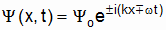. (9_9)

As it turns out, the wave function Y, first suggested by deBroglie and later given statistical meaning by Born, is generally complex. Thus the exponential expressions are ideal for manipulating Y mathematically.

10. The Infinite Square Well.

Let us suppose that a particle (say an electron) is trapped in a potential energy specified by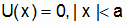, (10_1a)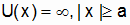. (10_1b)

By "trapped" we mean that the particle will never be found at any |x|>a. Thus according to Born,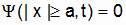. (10_2)

We expect at any moment to find the particle either (a) traveling in the positive x-direction or (b) traveling in the negative x-direction. Since U(x) is constantly zero inside the well, we can assume that there are 2 plane wave functions: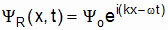, (10_3a)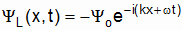, (10_3b)

where subscripts "R" and "L" stand for "Right" and "Left" respectively.

Why has Yo been preceded by a minus sign in Eq. 10_3b? Let us consider an electromagnetic wave. Such a wave undergoes a 180o phase reversal upon reflection, in effect making YR+YL=0 at x=+a. A more immediate reason in the present case has to do with the requirement that P be continuous in space. (We cannot have P differ by a finite amount between two points infinitesimally far apart.) Since, at x=a+dx, P=0, it follows that P(x=a) is essentially zero.Similarly at x=-a. Hence YL, the reflection of YR at x=a, must cancel out YR (and hence be 180o phase-changed) in order for the net wave function, Y=YR+YL, to equal zero. Similar remarks apply to x=-a.

Let us imagine that we are sitting at x=-a at time t=0. We shall say that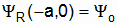, (10_4a)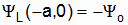. (10_4b)

If YR is to cancel YL at x=+a, then YR must rotate counterclockwise an integral number of half wavelengths in going from x=-a to x=+a. And YL must rotate clockwise an integral number of half wavelengths. If the number of half waves is 1, then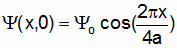. (10_5)

If the number of half waves is 2, then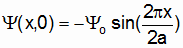, (10_6)

etc. The wavelengths, in descending order, are 4a, 2a, a, … Note that only discrete values of l fit into the well. And of course only discrete values of the particle energy, E, can be accommodated by the well.

What happens at times other than t=0? Consider Eq. 10_5. The cosine curve spins clockwise around the x-axis as time advances. In the lowest energy state (a half wave length fits in the well) It is somewhat like a playground jump rope being whirled by two children. But notice here that P(x) is constant in time. Such cases are referred to as stationary states.

Although YR and YL are simple plane waves, it is of fundamental importance to realize that Y, their sum at any given instant, is proportional to cosine or sine. Thus YY*=P is proportional to cosine2 or sine2. P is decidedly not a flat line as might classically be expected. P consists of alternating zeros and peaks. In the case of a macroscopic particle in a macroscopic-width well, the zeros and peaks may be much too close to be distinguished experimentally, and we measure a flat-line P. But as Bohr might say, the oscillations of P are there, whether or not we are able to discern them. Of course in the case of a microscopic particle in a microscopic well, the zeros and peaks are not only much more evident; they are also more significant.

Two important generalizations from the current discussion are that (1) all "elementary" Y’s must be added prior to calculating P, the probability density, and (2) particles bound to a finite region of space have only discrete energies.

11. Matter Waves.

In seeking to postulate a differential wave equation for matter waves, let us begin by considering light. According to deBroglie, the same equations apply to light particles (photons) and to material particles (e.g., electrons):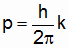, (11_1a)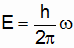. (11_1b)

In the case of light,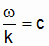. (11_2)

If we have a wave specified by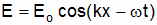, (11_3)

then this is a solution of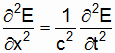. (11_4)

Eq. 11_4 is a natural consequence of Maxwell’s equations. (As previously mentioned, Maxwell was astute enough to recognize it as a wave equation.)

Now in the case of photons, the energy is proportional to the momentum: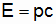. (11_5)

But in the case of electrons (for example), the kinetic energy is proportional to the square of the momentum: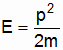. (11_6)

In other words, in the case of electrons whose total energy is kinetic,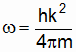. (11_7)

Let us suppose that we have a matter wave of the form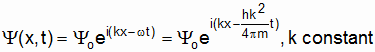. (11_8)

The second derivative w.r.t. x is proportional to k2: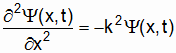. (11_9)

But it is the first derivative w.r.t. time that is also proportional to k2: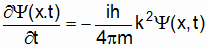. (11_10)

Thus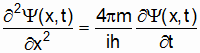. (11_11)

Theoretically Eq. 11_11 is the governing differential equation (a) when Eq. 11_8 is a solution, and (b) when Eq. 11_6 is the energy. More generally, any linear combination of equations with the form of Eq. 11_8 is a solution of Eq. 11_11.

Eq. 11_11 might be called the wave equation for matter waves. For reasons to be made clear shortly, it is written in the form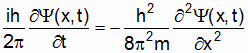. (11_12)

Now Eq. 11_6 is the energy of an electron with no potential energy. If the electron has a constant potential energy, Uo, in addition to its kinetic energy, then in lieu of Eq. 11_6 we would write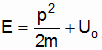. (11_13)

In such cases we would have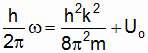, (11_14a)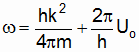. (11_14b)

The matter wave in this case would be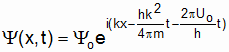. (11_15)

Again. (11_16)

But now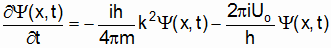. (11_17)

Algebraic manipulation produces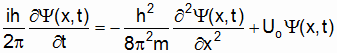. (11_18)

Eq. 11_18 is the wave equation for an electron with constant potential energy.

12. The Schroedinger Equation.

In the last section we derived the result. (12_1)

This is theoretically the governing matter-wave equation when a particle is in a constant potential energy.

It is of course a matter of interest when the potential energy is not constant. Most generally one wonders what the governing equation is when U is a function of both x and t. Schroedinger postulated that this equation is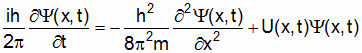. (12_2)

The test of this postulate would be whether or not the equation’s solutions agree with physical reality. Needless to say, its success has been spectacular!

A special case of great interest is when U is a function only of x. (This would be typical of a classically conservative system.) In that case the Schroedinger equation becomes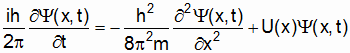(12_3)

We expect that both a solution’s amplitude and wave number might vary in x, say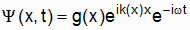. (12_4)

Or, defining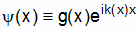, (12_5a)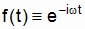, (12_5b)

we can say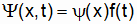. (12_6)

If we further define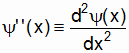, (12_7a)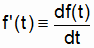, (12_7b)

then Eq. 12_3 can be written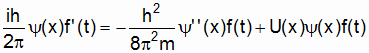. (12_8)

Dividing through by y(x)f(t) produces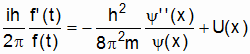. (12_9)

But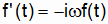. (12_10)

Thus Eq. 12_9 becomes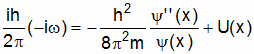. (12_11)

But according to deBroglie, wh/2p is just the total energy, E. Thus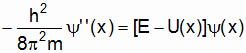. (12_12)

This ordinary differential equation in x is the time-independent Schroedinger equation. It is much easier to solve than Eq. 12_3. Since many systems are conservative in the classical sense, the time-independent equation is of great importance.

Note that, since e0=1, YY*=yy*. Thus P(x) follows directly from a solution of Eq. 12_12. It is perhaps worth reiterating that P is a function only of x in such cases … a condition generally referred to as a stationary state.

13. Concluding Remarks.

By now the reader is hopefully better positioned for a first course in quantum theory. Most introductory courses focus upon the Schroedinger equation (wave mechanics). But there is another formulation of the theory based on matrices, and introduced by Heisenberg, Born and others. Chances are that the first-course student will gain limited exposure to the matrix formulation. (The two formulations … wave mechanics and matrix theory … were shown by Schroedinger to be equivalent.)

Quantum theory has been described as the greatest intellectual achievement of the 20th century. Initially it addressed non-relativistic systems. But it was not long before Dirac and others extended it to relativistic regimes. More recently a different perspective into the behavior of minuscule systems … Quantum Electrodynamics (QED) … has entered the mix. (Students who go on to study QED will perhaps benefit from the spinning spirals model.)

To those who decide to forge ahead and take that first, introductory course, and particularly to those who plan to make a career in physics, the author wishes them smooth sailing. Many strange and intriguing new ideas await you. Enjoy the quantum world. You will be intellectually richer for the experience.